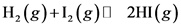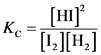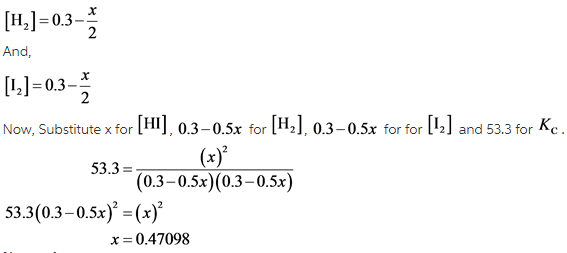# At a certain temperature, the equilibrium constant, Kc for this reaction is 53.3

#1

At a certain temperature, the equilibrium constant, Kc for this reaction is 53.3.
H2(g)+I2(g) = 2HI(g)

At this temperature, 0.300 mol of H2 and 0.300 mol of I2 were placed in a 1.00 L container to react. What concentration of HI is present at equilibrium?

Concepts and reason
The concept used to solve this problem is based on equilibrium constant.
The concentration of product or reactant present at equilibrium is calculated by the writing the formula of equilibrium constant.

Fundamentals
The equilibrium constant is the ratio of product’s concentration raised to power their stoichiometric coefficient to the reactant’s concentration raised to power their stoichiometric co-efficient.

The reaction is as follows:The equilibrium constant for this reaction is written as follows:The equilibrium constant is written by dividing the concentration of product raised to power their stochiometric coefficient and concentration of reactant raised to power their stoichiometric co-efficient.

Let the number of moles of HI formed is x. Then concentration of H2and I2 is written as follows:The concentration of HI present at equilibrium is 0.471 M.

The concentration of HI present at equilibrium is 0.237 M.